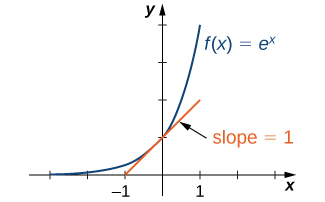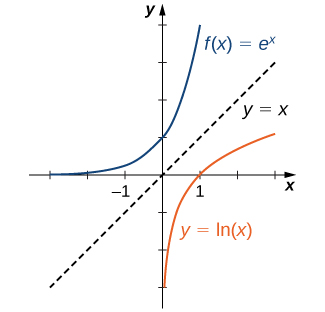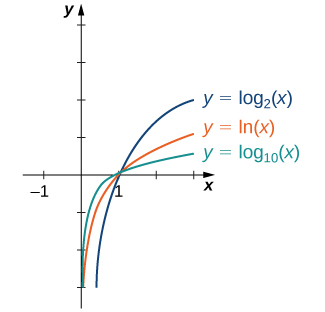$$\newcommand{\id}{\mathrm{id}}$$ $$\newcommand{\Span}{\mathrm{span}}$$ $$\newcommand{\kernel}{\mathrm{null}\,}$$ $$\newcommand{\range}{\mathrm{range}\,}$$ $$\newcommand{\RealPart}{\mathrm{Re}}$$ $$\newcommand{\ImaginaryPart}{\mathrm{Im}}$$ $$\newcommand{\Argument}{\mathrm{Arg}}$$ $$\newcommand{\norm}{\| #1 \|}$$ $$\newcommand{\inner}{\langle #1, #2 \rangle}$$ $$\newcommand{\Span}{\mathrm{span}}$$

# 1.9: Limit of Exponential Functions and Logarithmic Functions

$$\newcommand{\vecs}{\overset { \rightharpoonup} {\mathbf{#1}} }$$ $$\newcommand{\vecd}{\overset{-\!-\!\rightharpoonup}{\vphantom{a}\smash {#1}}}$$$$\newcommand{\id}{\mathrm{id}}$$ $$\newcommand{\Span}{\mathrm{span}}$$ $$\newcommand{\kernel}{\mathrm{null}\,}$$ $$\newcommand{\range}{\mathrm{range}\,}$$ $$\newcommand{\RealPart}{\mathrm{Re}}$$ $$\newcommand{\ImaginaryPart}{\mathrm{Im}}$$ $$\newcommand{\Argument}{\mathrm{Arg}}$$ $$\newcommand{\norm}{\| #1 \|}$$ $$\newcommand{\inner}{\langle #1, #2 \rangle}$$ $$\newcommand{\Span}{\mathrm{span}}$$ $$\newcommand{\id}{\mathrm{id}}$$ $$\newcommand{\Span}{\mathrm{span}}$$ $$\newcommand{\kernel}{\mathrm{null}\,}$$ $$\newcommand{\range}{\mathrm{range}\,}$$ $$\newcommand{\RealPart}{\mathrm{Re}}$$ $$\newcommand{\ImaginaryPart}{\mathrm{Im}}$$ $$\newcommand{\Argument}{\mathrm{Arg}}$$ $$\newcommand{\norm}{\| #1 \|}$$ $$\newcommand{\inner}{\langle #1, #2 \rangle}$$ $$\newcommand{\Span}{\mathrm{span}}$$

## Limit of Exponential Functions

Definition

A quantity grows linearly over time if it increases by a fixed amount with each time interval. A quantity decreases linearly over time if it decreases by a fixed amount with each time interval.

Example $$\PageIndex{1}$$:

If you start with $1000 and put$200 in a jar every month to save for a vacation, then every month the vacation savings grow by $200 and in x months you will have: Amount = 1000 + 200x Definition A quantity grows exponentially over time if it increases by a fixed percentage with each time interval. A quantity decays exponentially over time if it decreases by a fixed percentage with each time interval. Example $$\PageIndex{2}$$: If you start with a debt of$1000 and you are charged an annual interest rate of 24 percent (typical credit card interest rate) then how much will you owe after X months?

24 percent per year = 2 percent per month (this is how they convert it to a monthly interest rate)

EXPONENTIAL FUNCTION

For any real number $$x$$, an exponential function is a function with the form

$$f(x)=b^x$$

where

• $$b$$ is any positive real number such that $$b≠1$$.
• The domain of $$f$$ is all real numbers.
• The range of $$f$$ is all positive real numbers.
• The y-intercept is $$(0,1)$$,
• and the horizontal asymptote is $$y=0$$.

CHARACTERISTICS OF THE EXPONENTIAL FUNCTION

An exponential function with the form $$f(x)=b^x$$, $$b>0$$, $$b≠1$$,has these characteristics:

• one-to-one function
• horizontal asymptote: $$y=0$$
• domain: $$(–\infty, \infty)$$
• range: $$(0,\infty)$$
• x-intercept: none
• y-intercept: $$(0,1)$$
• increasing if $$b>1$$
• decreasing if $$b<1$$Rule: Laws of Exponents

For any constants $$a>0$$,$$b>0$$, and for all x and y,

1. $$b^x⋅b^y=b^{x+y}$$
2. $$\dfrac{b^x}{b^y}=b^{x−y}$$
3. $$(b^x)^y=b^{xy}$$
4. $$(ab)^x=a^xb^x$$
5. $$\dfrac{a^x}{b^x}=(\dfrac{a}{b})^x$$

Example $$\PageIndex{2}$$: Using the Laws of Exponents

Use the laws of exponents to simplify each of the following expressions.

1. $$\dfrac{(2x^{2/3})^3}{(4x^{−1/3})^2}$$
2. $$\dfrac{(x^3y^{−1})^2}{(xy^2)^{−2}}$$

Soution

a. We can simplify as follows:

$$\dfrac{(2x^{2/3})^3}{(4x^{−1/3})^2}$$=$$\dfrac{2^3(x^{2/3})^3}{4^2(x^{−1/3})^2}$$=$$\dfrac{8x^2}{16x^{−2/3}}$$=$$\dfrac{x^2x^{2/3}}{2}$$=$$\dfrac{x^{8/3}}{2}.$$

b. We can simplify as follows:

$$\dfrac{(x^3y^{−1})^2}{(xy^2)^{−2}}=\dfrac{(x3)^2(y^{−1})^2}{x−2(y^2)^{−2}}=\dfrac{x^6y^{−2}}{x^{−2}y^{−4}} =x^6x^2y^{−2}y^4=x^8y^2$$.

Exercise $$\PageIndex{2}$$:

Use the laws of exponents to simplify $$(6x^{−3}y^2)/(12x^{−4}y^5)$$.

Hint:

$$x^a/x^b=x^{a-b}$$

$$x/(2y^3)$$

## The Number e

A special type of exponential function appears frequently in real-world applications. To describe it, consider the following example of exponential growth, which arises from compounding interest in a savings account. Suppose a person invests $$P$$ dollars in a savings account with an annual interest rate $$r$$, compounded annually. The amount of money after 1 year is

$$A(1)=P+rP=P(1+r)$$.

The amount of money after $$2$$ years is

$$A(2)=A(1)+rA(1)=P(1+r)+rP(1+r)=P(1+r)^2$$.

More generally, the amount after $$t$$ years is

$$A(t)=P(1+r)^t$$.

If the money is compounded 2 times per year, the amount of money after half a year is

$$A(\dfrac{1}{2})=P+(\dfrac{r}{2})P=P(1+(\dfrac{r}{2}))$$.

The amount of money after $$1$$ year is

$$A(1)=A(\dfrac{1}{2})+(\dfrac{r}{2})A(\dfrac{1}{2})=P(1+\dfrac{r}{2})+\dfrac{r}{2}(P(1+\dfrac{r}{2}))=P(1+\dfrac{r}{2})^2.$$

After $$t$$ years, the amount of money in the account is

$$A(t)=P(1+\dfrac{r}{2})^{2t}$$.

More generally, if the money is compounded $$n$$ times per year, the amount of money in the account after $$t$$ years is given by the function

$$A(t)=P(1+\dfrac{r}{n})^{nt}.$$

What happens as $$n→∞?$$ To answer this question, we let $$m=n/r$$ and write

$$(1+\dfrac{r}{n})^{nt}=(1+\dfrac{1}{m})^{mrt},$$

and examine the behavior of $$(1+1/m)^m$$ as $$m→∞$$, using a table of values (Table).

 $$m$$ $$(1+\dfrac{1}{m})^m$$ 10 100 1000 10,000 100,000 1,000,000 2.5937 2.7048 2.71692 2.71815 2.718268 2.718280

Looking at this table, it appears that $$(1+1/m)^m$$ is approaching a number between $$2.7$$ and $$2.8$$ as $$m→∞$$. In fact, $$(1+1/m)^m$$ does approach some number as $$m→∞$$. We call this number $$e$$. To six decimal places of accuracy,

$$e≈2.718282$$.

The letter $$e$$ was first used to represent this number by the Swiss mathematician Leonhard Euler during the 1720s. Although Euler did not discover the number, he showed many important connections between $$e$$ and logarithmic functions. We still use the notation $$e$$ today to honor Euler’s work because it appears in many areas of mathematics and because we can use it in many practical applications.

Returning to our savings account example, we can conclude that if a person puts $$P$$ dollars in an account at an annual interest rate r, compounded continuously, then $$A(t)=Pe^{rt}$$. This function may be familiar. Since functions involving base e arise often in applications, we call the function $$f(x)=e^x$$ the natural exponential function. Not only is this function interesting because of the definition of the number $$e$$, but also, as discussed next, its graph has an important property.

Since $$e>1$$, we know ex is increasing on $$(−∞,∞)$$. In Figure, we show a graph of $$f(x)=e^x$$ along with a tangent line to the graph of at $$x=0$$. We give a precise definition of tangent line in the next chapter; but, informally, we say a tangent line to a graph of $$f$$ at $$x=a$$ is a line that passes through the point $$(a,f(a))$$ and has the same “slope” as $$f$$ at that point . The function $$f(x)=e^x$$ is the only exponential function $$b^x$$ with tangent line at $$x=0$$ that has a slope of 1. As we see later in the text, having this property makes the natural exponential function the most simple exponential function to use in many instances.Figure $$\PageIndex{3}$$: The graph of $$f(x)=e^x$$ has a tangent line with slope $$1$$ at $$x=0$$.

Example $$\PageIndex{3}$$: Compounding Interest

Suppose $$500$$ is invested in an account at an annual interest rate of $$r=5.5%$$, compounded continuously.

1. Let $$t$$ denote the number of years after the initial investment and A(t) denote the amount of money in the account at time $$t$$. Find a formula for $$A(t)$$.
2. Find the amount of money in the account after $$10$$ years and after $$20$$ years.

Solution

a. If $$P$$ dollars are invested in an account at an annual interest rate $$r$$, compounded continuously, then $$A(t)=Pe^{rt}$$. Here $$P=500$$ and $$r=0.055$$. Therefore, $$A(t)=500e^{0.055t}$$.

b. After $$10$$ years, the amount of money in the account is

$$A(10)=500e^{0.055⋅10}=500e^{0.55}≈866.63$$.

After $$20$$ years, the amount of money in the account is

$$A(20)=500e^{0.055⋅20}=500e^{1.1}≈1,502.08$$.

Exercise $$\PageIndex{3}$$

If $$750$$ is invested in an account at an annual interest rate of $$4%$$, compounded continuously, find a formula for the amount of money in the account after $$t$$ years. Find the amount of money after $$30$$ years.

Hint

$$A(t)=Pe^{rt}$$

(A(t)=750e^{0.04t}\). After $$30$$ years, there will be approximately $$2,490.09$$.

## Limit laws

$$\lim_{x\rightarrow \infty} b^x= \infty$$, if $$b>1$$.

$$\lim_{x\rightarrow -\infty} b^x= 0$$, if $$b>1$$.

$$\lim_{x\rightarrow \infty} b^x= 0$$, if $$0<b<1$$.

$$\lim_{x\rightarrow -\infty} b^x= \infty$$, if $$0<b<1$$.

Note

$$\lim_{x\rightarrow \infty} e^x= \infty$$.

$$\lim_{x\rightarrow -\infty} e^x= 0$$.

$$\lim_{x\rightarrow \infty} e^{-x}= 0$$.

$$\lim_{x\rightarrow -\infty} e^{-x}= \infty$$.

$$\lim\limits_{x\to 0} (1+x)^\frac1x = e$$

$$\lim\limits_{x\to 0} \frac{e^x-1}{x} = 1$$

## Logarithmic Functions

Using our understanding of exponential functions, we can discuss their inverses, which are the logarithmic functions. These come in handy when we need to consider any phenomenon that varies over a wide range of values, such as pH in chemistry or decibels in sound levels.

The exponential function $$f(x)=b^x$$ is one-to-one, with domain $$(−∞,∞)$$ and range $$(0,∞)$$. Therefore, it has an inverse function, called the logarithmic function with base $$b$$. For any $$b>0,b≠1$$, the logarithmic function with base b, denoted $$log_b$$, has domain $$(0,∞)$$ and range $$(−∞,∞)$$,and satisfies

$$log_b(x)=y$$ if and only if $$b^y=x$$.

For example,

$$log_2(8)=3$$ since $$2^3=8$$,

$$log_{10}(\dfrac{1}{100})=−2$$ since $$10^{−2}=\dfrac{1}{10^2}=\dfrac{1}{100}$$,

$$log_b(1)=0$$ since $$b^0=1$$ for any base $$b>0$$.

Furthermore, since $$y=log_b(x)$$ and $$y=b^x$$ are inverse functions,

$\log_b(b^x)=x$

and

$b^{\log_b(x)}=x.$

The most commonly used logarithmic function is the function $$log_e$$. Since this function uses natural e as its base, it is called the natural logarithm. Here we use the notation $$\ln (x)$$ or $$\ln x$$ to mean $$log_e(x)$$. For example,

$\ln (e)=log_e(e)=1, \ln (e^3)=log_e(e^3)=3, \ln (1)=log_e(1)=0.$

Since the functions $$f(x)=e^x$$ and $$g(x)=\ln (x)$$ are inverses of each other,

$$\ln (e^x)=x$$ and $$e^{\ln x}=x$$,

and their graphs are symmetric about the line $$y=x$$ (Figure).Figure $$\PageIndex{4}$$: The functions $$y=e^x$$ and $$y=\ln (x)$$ are inverses of each other, so their graphs are symmetric about the line $$y=x$$.

In general, for any base $$b>0$$,$$b≠1$$, the function $$g(x)=log_b(x)$$ is symmetric about the line $$y=x$$ with the function $$f(x)=b^x$$. Using this fact and the graphs of the exponential functions, we graph functions $$log_b$$ for several values of b>1 (Figure).Figure $$\PageIndex{5}$$: Graphs of $$y=log_b(x)$$ are depicted for $$b=2,e,10$$.

Before solving some equations involving exponential and logarithmic functions, let’s review the basic properties of logarithms.

Properties of Logarithms

If $$a,b,c>0,b≠1$$, and $$r$$ is any real number, then

1. $$log_b(ac)=log_b(a)+log_b(c)$$ (Product property)
2. $$log_b(\dfrac{a}{c})=log_b(a)−log_b(c)$$ (Quotient property)
3. $$log_b(a^r)=rlog_b(a)$$ (Power property)

Example $$\PageIndex{4}$$: Solving Equations Involving Exponential Functions

Solve each of the following equations for $$x$$.

1. $$5^x=2$$
2. $$e^x+6e^{−x}=5$$

Solution

a. Applying the natural logarithm function to both sides of the equation, we have

$$\ln 5^x=\ln 2$$.

Using the power property of logarithms,

$$x\ln 5=\ln 2.$$

Therefore, $$x=\ln 2/\ln 5.$$

b. Multiplying both sides of the equation by $$e^x$$,we arrive at the equation

$$e^{2x}+6=5e^x$$.

Rewriting this equation as

$$e^{2x}−5e^x+6=0$$,

we can then rewrite it as a quadratic equation in $$e^x$$:

$$(e^x)^2−5(e^x)+6=0.$$

Now we can solve the quadratic equation. Factoring this equation, we obtain

$$(e^x−3)(e^x−2)=0.$$

Therefore, the solutions satisfy $$e^x=3$$ and $$e^x=2$$. Taking the natural logarithm of both sides gives us the solutions $$x=\ln 3,\ln 2$$.

Exercise $$\PageIndex{4}$$

Solve $$e^{2x}/(3+e^{2x})=1/2$$.

Hint

First solve the equation for $$e^{2x}$$

$$x=\dfrac{\ln 3}{2}$$.

Example $$\PageIndex{5}$$: Solving Equations Involving Logarithmic Functions

Solve each of the following equations for $$x$$.

1. $$\ln (\dfrac{1}{x})=4$$
2. $$log_10\sqrt{x}+log_10x=2$$
3. $$\ln (2x)−3\ln (x^2)=0$$

Solution

a. By the definition of the natural logarithm function,

$$\ln (\dfrac{1}{x})=4$$ if and only if $$e^4=\dfrac{1}{x}$$.

Therefore, the solution is $$x=1/e^4$$.

b. Using the product and power properties of logarithmic functions, rewrite the left-hand side of the equation as

$$log_10\dfrac{x}+log_10x=log_10x\dfrac{x}=log_10x^{3/2}=\dfrac{3}{2}log_10x$$.

Therefore, the equation can be rewritten as

$$\dfrac{3}{2}log_10x=2$$ or $$log_10x=\dfrac{4}{3}$$.

The solution is $$x=10^{4/3}=10\dfrac{10}$$.

c. Using the power property of logarithmic functions, we can rewrite the equation as $$\ln (2x)−\ln (x^6)=0$$.

Using the quotient property, this becomes

$$\ln (\dfrac{2}{x^5})=0$$

Therefore, $$2/x^5=1$$, which implies $$x=\sqrt{2}$$. We should then check for any extraneous solutions.

Exercise $$\PageIndex{5}$$

Solve $$\ln (x^3)−4\ln (x)=1$$.

Hint

First use the power property, then use the product property of logarithms.

$$x=\dfrac{1}{e}$$

When evaluating a logarithmic function with a calculator, you may have noticed that the only options are $$log_10$$ or log, called the common logarithm, or \ln , which is the natural logarithm. However, exponential functions and logarithm functions can be expressed in terms of any desired base $$b$$. If you need to use a calculator to evaluate an expression with a different base, you can apply the change-of-base formulas first. Using this change of base, we typically write a given exponential or logarithmic function in terms of the natural exponential and natural logarithmic functions.

Rule: Change-of-Base Formulas

Let $$a>0,b>0$$, and $$a≠1,b≠1$$.

1. $$a^x=b^{xlog_ba}$$ for any real number $$x$$.

If $$b=e$$, this equation reduces to $$a^x=e^{xlog_ea}=e^{x\ln a}$$.

2. $$log_ax=\dfrac{log_bx}{log_ba}$$ for any real number $$x>0$$.

If $$b=e$$, this equation reduces to $$log_ax=\dfrac{\ln x}{\ln a}$$.

Proof

For the first change-of-base formula, we begin by making use of the power property of logarithmic functions. We know that for any base $$b>0,b≠1$$, $$log_b(a^x)=xlog_ba$$. Therefore,

$$b^{log_b(a^x)}$$=$$b^{xlog_ba}$$.

In addition, we know that $$b^x$$ and $$log_b(x)$$ are inverse functions. Therefore,

$$b^{log_b(a^x)}=a^x$$.

Combining these last two equalities, we conclude that $$a^x=b^{xlog_ba}$$.

To prove the second property, we show that

$$(log_ba)⋅(log_ax)=log_bx.$$

Let $$u=log_ba,v=log_ax$$, and $$w=log_bx$$. We will show that $$u⋅v=w$$. By the definition of logarithmic functions, we know that $$b^u=a,a^v=x$$, and $$b^w=x$$.From the previous equations, we see that

$$b^{uv}=(b^u)^v=a^v=x=b^w.$$

Therefore, $$b^{uv}=b^w$$. Since exponential functions are one-to-one, we can conclude that $$u⋅v=w$$.

$$\square$$

Example $$\PageIndex{6}$$: Changing Bases

Use a calculating utility to evaluate $$log_37$$ with the change-of-base formula presented earlier.

Solution

Use the second equation with $$a=3$$ and $$e=3$$: $$log_37=\dfrac{\ln 7}{\ln 3}≈1.77124$$.

Exercise $$\PageIndex{6}$$

Use the change-of-base formula and a calculating utility to evaluate $$log_46$$.

Hint

Use the change of base to rewrite this expression in terms of expressions involving the natural logarithm function.

$$1.29248$$

Example $$\PageIndex{7}$$: The Richter Scale for EarthquakesFigure $$\PageIndex{6}$$: (credit: modification of work by Robb Hannawacker, NPS)

In 1935, Charles Richter developed a scale (now known as the Richter scale) to measure the magnitude of an earthquake. The scale is a base-10 logarithmic scale, and it can be described as follows: Consider one earthquake with magnitude $$R_1$$ on the Richter scale and a second earthquake with magnitude $$R_2$$ on the Richter scale. Suppose $$R_1>R_2$$, which means the earthquake of magnitude $$R_1$$ is stronger, but how much stronger is it than the other earthquake? A way of measuring the intensity of an earthquake is by using a seismograph to measure the amplitude of the earthquake waves. If $$A_1$$ is the amplitude measured for the first earthquake and $$A_2$$ is the amplitude measured for the second earthquake, then the amplitudes and magnitudes of the two earthquakes satisfy the following equation:

$$R_1−R_2=log_{10}(\dfrac{A1}{A2})$$.

Consider an earthquake that measures 8 on the Richter scale and an earthquake that measures 7 on the Richter scale. Then,

$$8−7=log_{10}(\dfrac{A1}{A2})$$.

Therefore,

$$log_{10}(\dfrac{A1}{A2})=1$$,

which implies $$A_1/A_2=10$$ or $$A_1=10A_2$$. Since $$A_1$$ is 10 times the size of $$A_2$$, we say that the first earthquake is 10 times as intense as the second earthquake. On the other hand, if one earthquake measures 8 on the Richter scale and another measures 6, then the relative intensity of the two earthquakes satisfies the equation

$$log_{10}(\dfrac{A1}{A2})=8−6=2$$.

Therefore, $$A_1=100A_2$$.That is, the first earthquake is 100 times more intense than the second earthquake.

How can we use logarithmic functions to compare the relative severity of the magnitude 9 earthquake in Japan in 2011 with the magnitude 7.3 earthquake in Haiti in 2010?

Solution

To compare the Japan and Haiti earthquakes, we can use an equation presented earlier:

$$9−7.3=log_{10}(\dfrac{A1}{A2})$$.

Therefore, $$A_1/A_2=10^{1.7}$$, and we conclude that the earthquake in Japan was approximately 50 times more intense than the earthquake in Haiti.

Exercise $$\PageIndex{7}$$

Compare the relative severity of a magnitude $$8.4$$ earthquake with a magnitude $$7.4$$ earthquake.

Hint

$$R_1−R_2=log_{10}(A1/A2)$$.

The magnitude $$8.4$$ earthquake is roughly $$10$$ times as severe as the magnitude $$7.4$$ earthquake.

Example $$\PageIndex{8}$$: Determining End Behavior for a Transcendental Function

Find the limits as $$x→∞$$ and $$x→−∞$$ for $$f(x)=\frac{(2+3e^x)}{(7−5ex^)}$$ and describe the end behavior of $$f.$$

Solution

To find the limit as $$x→∞,$$ divide the numerator and denominator by $$e^x$$:

$$\displaystyle \lim_{x→∞}f(x)=\lim_{x→∞}\frac{2+3e^x}{7−5e^x}$$

$$=\lim_{x→∞}\frac{(2/e^x)+3}{(7/e^x)−5.}$$

As shown in Figure, $$e^x→∞$$ as $$x→∞.$$ Therefore,

$$\displaystyle \lim_{x→∞}\frac{2}{e^x}=0=\lim_{x→∞}\frac{7}{e^x}$$.

We conclude that $$\displaystyle \lim_{x→∞f}(x)=−\frac{3}{5}$$, and the graph of $$f$$ approaches the horizontal asymptote $$y=−\frac{3}{5}$$ as $$x→∞.$$ To find the limit as $$x→−∞$$, use the fact that $$e^x→0$$ as $$x→−∞$$ to conclude that $$\displaystyle \lim_{x→∞}f(x)=\frac{2}{7}$$, and therefore the graph of approaches the horizontal asymptote $$y=\frac{2}{7}$$ as $$x→−∞$$.

Exercise $$\PageIndex{8}$$

Find the limits as $$x→∞$$ and $$x→−∞$$ for $$f(x)=\frac{(3e^x−4)}{(5e^x+2).}$$

Hint

$$\displaystyle \lim_{x→∞}e^x=∞$$ and $$im_{x→∞}e^x=0.$$

$$\displaystyle \lim_{x→∞}f(x)=\frac{3}{5}, \lim_{x→−∞}f(x)=−2$$

## Key Concepts

• The exponential function $$y=b^x$$ is increasing if $$b>1$$ and decreasing if $$0<b<1$$. Its domain is $$(−∞,∞)$$ and its range is $$(0,∞)$$.
• The logarithmic function $$y=log_b(x)$$ is the inverse of $$y=b^x$$. Its domain is $$(0,∞)$$ and its range is $$(−∞,∞)$$.
• The natural exponential function is $$y=e^x$$ and the natural logarithmic function is $$y=\ln x=log_ex$$.
• Given an exponential function or logarithmic function in base $$a$$, we can make a change of base to convert this function to any base $$b>0$$, $$b≠1$$. We typically convert to base $$e$$.

## Glossary

base
the number $$b$$ in the exponential function $$f(x)=b^x$$ and the logarithmic function $$f(x)=log_bx$$
exponent
the value $$x$$ in the expression $$b^x$$
natural exponential function
the function $$f(x)=e^x$$
natural logarithm
the function $$\ln x=log_ex$$
number e
as $$m$$ gets larger, the quantity $$(1+(1/m)^m$$ gets closer to some real number; we define that real number to be e; the value of e is approximately $$2.718282$$